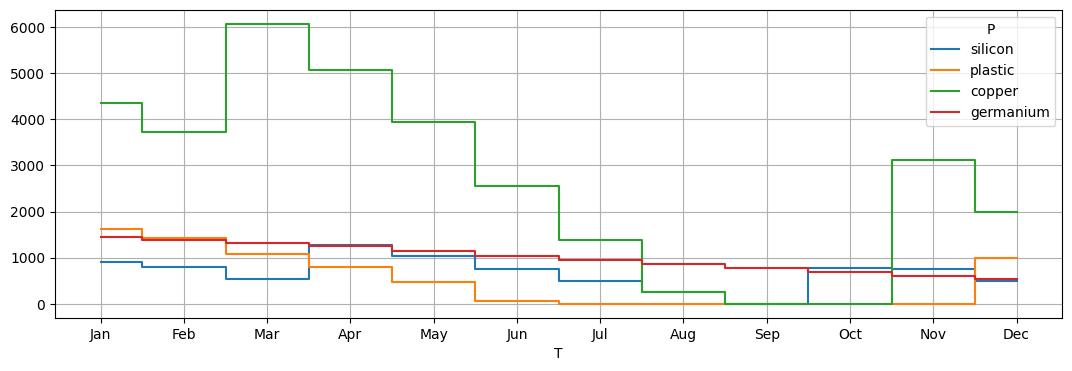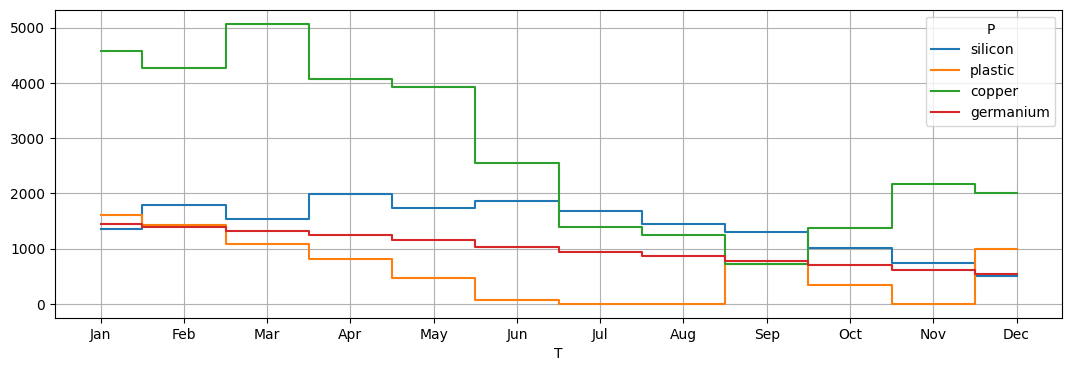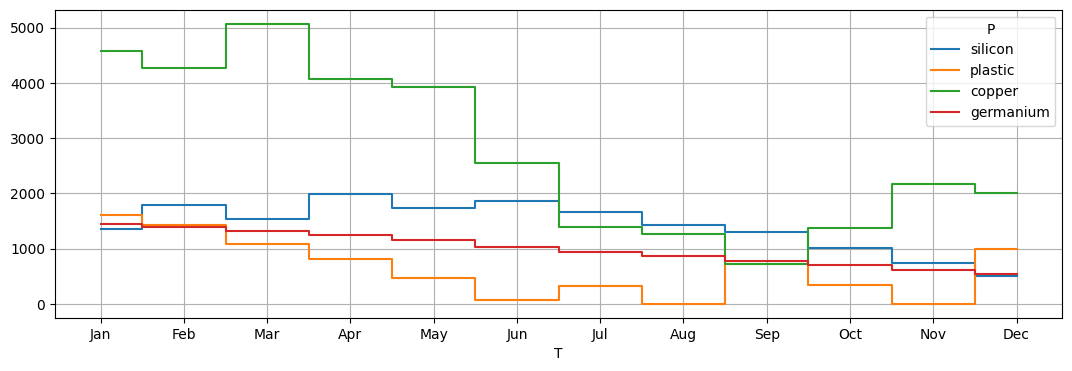# BIM production using demand forecasts#

# install dependencies and select solver
%pip install -q amplpy numpy matplotlib

SOLVER = "highs"

from amplpy import AMPL, ampl_notebook

ampl = ampl_notebook(
modules=["highs"],  # modules to install
)  # instantiate AMPL object and register magics

Using default Community Edition License for Colab. Get yours at: https://ampl.com/ce
Licensed to AMPL Community Edition License for the AMPL Model Colaboratory (https://colab.ampl.com).


## The problem: Optimal material acquisition and production planning using demand forecasts#

This example is a continuation of the BIM chip production problem illustrated here. Recall hat BIM produces logic and memory chips using copper, silicon, germanium, and plastic and that each chip requires the following quantities of raw materials:

chip

copper

silicon

germanium

plastic

logic

0.4

1

-

1

memory

0.2

-

1

1

BIM needs to carefully manage the acquisition and inventory of these raw materials based on the forecasted demand for the chips. Data analysis led to the following prediction of monthly demands:

chip

Jan

Feb

Mar

Apr

May

Jun

Jul

Aug

Sep

Oct

Nov

Dec

logic

88

125

260

217

238

286

248

238

265

293

259

244

memory

47

62

81

65

95

118

86

89

82

82

84

66

At the beginning of the year, BIM has the following stock:

copper

silicon

germanium

plastic

480

1000

1500

1750

The company would like to have at least the following stock at the end of the year:

copper

silicon

germanium

plastic

200

500

500

1000

Each raw material can be acquired at each month, but the unit prices vary as follows:

product

Jan

Feb

Mar

Apr

May

Jun

Jul

Aug

Sep

Oct

Nov

Dec

copper

1

1

1

2

2

3

3

2

2

1

1

2

silicon

4

3

3

3

5

5

6

5

4

3

3

5

germanium

5

5

5

3

3

3

3

2

3

4

5

6

plastic

0.1

0.1

0.1

0.1

0.1

0.1

0.1

0.1

0.1

0.1

0.1

0.1

The inventory is limited by a capacity of a total of 9000 units per month, regardless of the type of material of products in stock. The holding costs of the inventory are 0.05 per unit per month regardless of the material type. Due to budget constraints, BIM cannot spend more than 5000 per month on acquisition.

BIM aims at minimizing the acquisition and holding costs of the materials while meeting the required quantities for production. The production is made to order, meaning that no inventory of chips is kept.

Let us model the material acquisition planning and solve it optimally based on the forecasted chip demand above.

Let us first import both the price and forecast chip demand as Pandas dataframes.

import numpy as np
import matplotlib.pyplot as plt
from io import StringIO
import pandas as pd

demand_data = """chip,Jan,Feb,Mar,Apr,May,Jun,Jul,Aug,Sep,Oct,Nov,Dec
logic,88,125,260,217,238,286,248,238,265,293,259,244
memory,47,62,81,65,95,118,86,89,82,82,84,66"""
price_data = """product,Jan,Feb,Mar,Apr,May,Jun,Jul,Aug,Sep,Oct,Nov,Dec
copper,1,1,1,2,2,3,3,2,2,1,1,2
silicon,4,3,3,3,5,5,6,5,4,3,3,5
germanium,5,5,5,3,3,3,3,2,3,4,5,6
plastic,0.1,0.1,0.1,0.1,0.1,0.1,0.1,0.1,0.1,0.1,0.1,0.1"""

display(demand_chips)

price

Jan Feb Mar Apr May Jun Jul Aug Sep Oct Nov Dec
chip
logic 88 125 260 217 238 286 248 238 265 293 259 244
memory 47 62 81 65 95 118 86 89 82 82 84 66
Jan Feb Mar Apr May Jun Jul Aug Sep Oct Nov Dec
product
copper 1.0 1.0 1.0 2.0 2.0 3.0 3.0 2.0 2.0 1.0 1.0 2.0
silicon 4.0 3.0 3.0 3.0 5.0 5.0 6.0 5.0 4.0 3.0 3.0 5.0
germanium 5.0 5.0 5.0 3.0 3.0 3.0 3.0 2.0 3.0 4.0 5.0 6.0
plastic 0.1 0.1 0.1 0.1 0.1 0.1 0.1 0.1 0.1 0.1 0.1 0.1

We can also add a small dataframe with the consumptions and obtain the monthly demand for each raw material using a simple matrix multiplication.

use = dict()
use["logic"] = {"silicon": 1, "plastic": 1, "copper": 4}
use["memory"] = {"germanium": 1, "plastic": 1, "copper": 2}
use = pd.DataFrame.from_dict(use).fillna(0).astype(int)
display(use)

demand = use.dot(demand_chips)
demand

logic memory
silicon 1 0
plastic 1 1
copper 4 2
germanium 0 1
Jan Feb Mar Apr May Jun Jul Aug Sep Oct Nov Dec
silicon 88 125 260 217 238 286 248 238 265 293 259 244
plastic 135 187 341 282 333 404 334 327 347 375 343 310
copper 446 624 1202 998 1142 1380 1164 1130 1224 1336 1204 1108
germanium 47 62 81 65 95 118 86 89 82 82 84 66

## The optimization model#

Define the set of raw material $$P=\{\text{copper},\text{silicon},\text{germanium},\text{plastic}\}$$ and $$T$$ the set of the $$12$$ months of the year. Let

• $$x_{pt} \geq 0$$ be the variable describing the amount of raw material $$p \in P$$ acquired in month $$t \in T$$;

• $$s_{pt} \geq 0$$ be the variable describing the amount of raw material $$p \in P$$ left in stock at the end of month $$t \in T$$. Note that these values are uniquely determined by the $$x$$ variables, but we keep these additional variables to ease the modeling.

The total cost is the objective function of our optimal acquisition and production problem. If $$\pi_{pt}$$ is the unit price of product $$p \in P$$ in month $$t \in T$$ and $$h_{pt}$$ the unit holding costs (which happen to be constant) we can express the total cost as:

$\sum_{p\in P}\sum_{t \in T}\pi_{pt}x_{pt} + \sum_{p\in P}\sum_{t \in T} h_{pt} s_{pt}.$

Let us now focus on the constraints. If $$\beta \geq 0$$ denotes the monthly acquisition budget, the budget constraint can be expressed as:

$\sum_{p\in P} \pi_{pt}x_{pt} \leq \beta \quad \forall t \in T.$

Further, we constrain the inventory to be always the storage capacity $$\ell \geq 0$$ using:

$\sum_{p\in P} s_{pt} \leq \ell \quad \forall t \in T.$

Next, we add another constraint to fix the value of the variables $$s_{pt}$$ by balancing the acquired amounts with the previous inventory and the demand $$\delta_{pt}$$ which for each month is implied by the total demand for the chips of both types. Note that $$t-1$$ is defined as the initial stock when $$t$$ is the first period, that is \texttt{January}. This can be obtained with additional variables $$s$$ made equal to those values or with a rule that specializes, as in the code below.

$x_{pt} + s_{p,t-1} = \delta_{pt} + s_{pt} \quad \forall p \in P, t \in T.$

Finally, we capture the required minimum inventory levels in December with the constraint.

$s_{p \textrm{Dec}} \geq \Omega_p \quad \forall p \in P,$

where $$(\Omega_p)_{p \in P}$$ is the vector with the desired end inventories.

Here is the AMPL implementation of this LP.

%%writefile BIMProductAcquisitionAndInventory.mod

set T ordered;
set P;

var x{P, T} >= 0;
var s{P, T} >= 0;

param pi{P, T};
param h{P, T} default 0.05;
param delta{P, T};
param existing{P};
param desired{P};
param month_budget;
param stock_limit;

var acquisition_cost = sum{p in P, t in T} pi[p,t] * x[p,t];
var inventory_cost = sum{p in P, t in T} h[p,t] * s[p,t];

minimize total_cost: acquisition_cost + inventory_cost;

s.t. balance {p in P, t in T}:
(if t == first(T) then
existing[p] + x[p,t]
else
x[p,t] + s[p,prev(t)])
== delta[p,t] + s[p,t];
s.t. finish {p in P}: s[p, last(T)] >= desired[p];
s.t. inventory {t in T}: sum{p in P} s[p,t] <= stock_limit;
s.t. budget {t in T}: sum{p in P} pi[p,t] * x[p,t] <= month_budget;

Overwriting BIMProductAcquisitionAndInventory.mod

def ShowTableOfAmplVariables(m, X):
P = m.set["P"].to_list()
T = m.set["T"].to_list()

df = pd.DataFrame(m.var[X].to_list(), columns=["P", "T", "values"]).round(
decimals=2
)
df = df.pivot(index="P", columns="T", values="values")
df = df.reindex(P)
df = df[T]

return df

def BIMProductAcquisitionAndInventory(
demand, acquisition_price, existing, desired, stock_limit, month_budget
):
m = AMPL()
m.set["T"] = list(demand.columns)
m.set["P"] = demand.index.values
m.param["pi"] = acquisition_price
m.param["delta"] = demand
m.param["existing"] = existing
m.param["desired"] = desired
m.param["month_budget"] = month_budget
m.param["stock_limit"] = stock_limit

return m


We now can create an instance of the model using the provided data and solve it.

budget = 5000
m = BIMProductAcquisitionAndInventory(
demand,
price,
{"silicon": 1000, "germanium": 1500, "plastic": 1750, "copper": 4800},
{"silicon": 500, "germanium": 500, "plastic": 1000, "copper": 2000},
9000,
budget,
)

m.option["solver"] = SOLVER
m.solve()

print("\nThe optimal amount of raw materials to acquire in each month is:")
display(ShowTableOfAmplVariables(m, "x"))
print("\nThe corresponding optimal stock levels in each months are:")
stock = ShowTableOfAmplVariables(m, "s")
display(stock)
print("\nThe stock levels can be visualized as follows")
stock.T.plot(drawstyle="steps-mid", grid=True, figsize=(13, 4))
plt.xticks(np.arange(len(stock.columns)), stock.columns)
plt.show()

HiGHS 1.5.3: HiGHS 1.5.3: optimal solution; objective 21152.655
29 simplex iterations
0 barrier iterations

The optimal amount of raw materials to acquire in each month is:

T Jan Feb Mar Apr May Jun Jul Aug Sep Oct Nov Dec
P
silicon 0.0 0.0 0.0 965.0 0.0 0.0 0.0 0.0 0.0 1078.1 217.9 0.0
plastic 0.0 0.0 0.0 0.0 0.0 0.0 266.0 327.0 347.0 375.0 343.0 1310.0
copper 0.0 0.0 3548.0 0.0 0.0 0.0 0.0 0.0 962.0 1336.0 4312.0 0.0
germanium 0.0 0.0 0.0 0.0 0.0 0.0 0.0 0.0 0.0 0.0 0.0 0.0
The corresponding optimal stock levels in each months are:

T Jan Feb Mar Apr May Jun Jul Aug Sep Oct Nov Dec
P
silicon 912.0 787.0 527.0 1275.0 1037.0 751.0 503.0 265.0 0.0 785.1 744.0 500.0
plastic 1615.0 1428.0 1087.0 805.0 472.0 68.0 0.0 0.0 0.0 0.0 0.0 1000.0
copper 4354.0 3730.0 6076.0 5078.0 3936.0 2556.0 1392.0 262.0 0.0 0.0 3108.0 2000.0
germanium 1453.0 1391.0 1310.0 1245.0 1150.0 1032.0 946.0 857.0 775.0 693.0 609.0 543.0
The stock levels can be visualized as followsHere is a different solution corresponding to the situation where the budget is much lower, namely 2000.

budget = 2000
m = BIMProductAcquisitionAndInventory(
demand,
price,
{"silicon": 1000, "germanium": 1500, "plastic": 1750, "copper": 4800},
{"silicon": 500, "germanium": 500, "plastic": 1000, "copper": 2000},
9000,
budget,
)

m.option["solver"] = SOLVER
m.solve()

print("\nThe optimal amount of raw materials to acquire in each month is:")
display(ShowTableOfAmplVariables(m, "x"))
print("\nThe corresponding optimal stock levels in each months are:")
stock = ShowTableOfAmplVariables(m, "s")
display(stock)
print("\nThe stock levels can be visualized as follows")
stock.T.plot(drawstyle="steps-mid", grid=True, figsize=(13, 4))
plt.xticks(np.arange(len(stock.columns)), stock.columns)
plt.show()

HiGHS 1.5.3: HiGHS 1.5.3: optimal solution; objective 25908.12917
44 simplex iterations
0 barrier iterations

The optimal amount of raw materials to acquire in each month is:

T Jan Feb Mar Apr May Jun Jul Aug Sep Oct Nov Dec
P
silicon 444.67 559.0 0.0 666.67 0.0 400.0 65.05 0.00 125.62 0.0 0.0 0.0
plastic 0.00 0.0 0.0 0.00 0.0 0.0 266.00 327.00 1065.00 0.0 0.0 1310.0
copper 221.33 323.0 2000.0 0.00 1000.0 0.0 0.00 983.65 695.52 2000.0 2000.0 934.5
germanium 0.00 0.0 0.0 0.00 0.0 0.0 0.00 0.00 0.00 0.0 0.0 0.0
The corresponding optimal stock levels in each months are:

T Jan Feb Mar Apr May Jun Jul Aug Sep Oct Nov Dec
P
silicon 1356.67 1790.67 1530.67 1980.33 1742.33 1856.33 1673.38 1435.38 1296.0 1003.0 744.0 500.0
plastic 1615.00 1428.00 1087.00 805.00 472.00 68.00 0.00 0.00 718.0 343.0 0.0 1000.0
copper 4575.33 4274.33 5072.33 4074.33 3932.33 2552.33 1388.33 1241.98 713.5 1377.5 2173.5 2000.0
germanium 1453.00 1391.00 1310.00 1245.00 1150.00 1032.00 946.00 857.00 775.0 693.0 609.0 543.0
The stock levels can be visualized as followsLooking at the two optimal solutions corresponding to different budgets, we can note that:

• The budget is not limitative;

• With the initial budget of 5000 the solution remains integer;

• Lowering the budget to 2000 forces acquiring fractional quantities;

• Lower values of the budget end up making the problem infeasible.

### A more parsimonious model#

We can create a more parsimonious model with fewer variabels by getting rid of the auxiliary variables $$s_{pt}$$. Here is the corresponding implementation in AMPL:

%%writefile BIMProductAcquisitionAndInventory_v2.mod

set T ordered;
set P;
param pi{P, T};
param h{P, T} default 0.05;
param delta{P, T};

param existing{P};
param desired{P};

param month_budget;
param stock_limit;
var x{P,T} >= 0;
param acquisition_price{P,T};
var s{p in P, t in T} = if t == first(T) then
existing[p] + x[p,t] - delta[p,t]
else
x[p,t] + s[p,prev(t)] - delta[p,t];
s.t. non_negative_stock {p in P, t in T}: s[p,t] >= 0;
var acquisition_cost = sum{p in P, t in T} pi[p,t] * x[p,t];
var inventory_cost = sum{p in P, t in T} h[p,t] * s[p,t];
minimize total_cost: acquisition_cost + inventory_cost;
s.t. finish {p in P}: s[p,last(T)] >= desired[p];
s.t. inventory {t in T}: sum{p in P} s[p,t] <= stock_limit;
s.t. budget {t in T}: sum{p in P} pi[p,t] * x[p,t] <= month_budget;

Overwriting BIMProductAcquisitionAndInventory_v2.mod

def BIMProductAcquisitionAndInventory_v2(
demand, acquisition_price, existing, desired, stock_limit, month_budget
):
m = AMPL()

m.set["T"] = list(demand.columns)
m.set["P"] = demand.index.values

m.param["pi"] = acquisition_price
m.param["delta"] = demand
m.param["existing"] = existing
m.param["desired"] = desired
m.param["month_budget"] = month_budget
m.param["stock_limit"] = stock_limit

return m

m = BIMProductAcquisitionAndInventory_v2(
demand,
price,
{"silicon": 1000, "germanium": 1500, "plastic": 1750, "copper": 4800},
{"silicon": 500, "germanium": 500, "plastic": 1000, "copper": 2000},
9000,
2000,
)

m.option["solver"] = SOLVER
m.solve()

print("\nThe optimal amount of raw materials to acquire in each month is:")
display(ShowTableOfAmplVariables(m, "x"))
print("\nThe corresponding optimal stock levels in each months are:")
stock = ShowTableOfAmplVariables(m, "s")
display(stock)
print("\nThe stock levels can be visualized as follows")
stock.T.plot(drawstyle="steps-mid", grid=True, figsize=(13, 4))
plt.xticks(np.arange(len(stock.columns)), stock.columns)
plt.show()

HiGHS 1.5.3: HiGHS 1.5.3: optimal solution; objective 25908.12917
21 simplex iterations
0 barrier iterations

The optimal amount of raw materials to acquire in each month is:

T Jan Feb Mar Apr May Jun Jul Aug Sep Oct Nov Dec
P
silicon 444.67 559.0 0.0 666.67 0.0 400.0 56.88 0.0 133.79 0.0 0.0 0.0
plastic 0.00 0.0 0.0 0.00 0.0 0.0 593.00 0.0 1065.00 0.0 0.0 1310.0
copper 221.33 323.0 2000.0 0.00 1000.0 0.0 0.00 1000.0 679.17 2000.0 2000.0 934.5
germanium 0.00 0.0 0.0 0.00 0.0 0.0 0.00 0.0 0.00 0.0 0.0 0.0
The corresponding optimal stock levels in each months are:

T Jan Feb Mar Apr May Jun Jul Aug Sep Oct Nov Dec
P
silicon 1356.67 1790.67 1530.67 1980.33 1742.33 1856.33 1665.21 1427.21 1296.0 1003.0 744.0 500.0
plastic 1615.00 1428.00 1087.00 805.00 472.00 68.00 327.00 0.00 718.0 343.0 0.0 1000.0
copper 4575.33 4274.33 5072.33 4074.33 3932.33 2552.33 1388.33 1258.33 713.5 1377.5 2173.5 2000.0
germanium 1453.00 1391.00 1310.00 1245.00 1150.00 1032.00 946.00 857.00 775.0 693.0 609.0 543.0
The stock levels can be visualized as follows